## Sunday, October 30, 2016

### Linear Gaussian Quadratic Control System

For the last year when I've had time, I have been developing an entirely new control scheme (at least as far as I know for the open source community) for multi rotors that replaces PID by expanding upon Tau Labs System Identification (see the mathematical description here).

The way linear quadratic control (LQG) works is by simultaneously estimating additional properties in the system we cannot directly measure (in this case the instantaneous torque and true rate of rotation) and the using those to control the system. I had previously experimented with something a bit ad hoc like this in the past.

## System Identification

A while ago Korken proposed a nice EKF that would extract a few critical properties of the system (primarily the 'gain' of each axis and the time constant of the motors). I wrote up an implementation of this and we got it running and measuring reasonable values. Based on this, I came up with some analytic approaches to optimize the PID control parameters based on these estimated system properties.

More specifically we use a model with several parameters that are specific to each frame $\beta_1$, $\beta_2$, $\tau$. The latency of the ESC/Motor/Prop is captured by $\tau$ and is one of the most performance limiting factors in a frame. For roll and pitch only $\beta_1$ is used and corresponds to the strength of that control output. For yaw there is a component that has no latency -- $\beta_2$ -- and that corresponds to directly generating a reaction force torque by accelerating the props.

$\dot \theta = \omega$
$\dot \omega = \beta_1 \cdot \nu + \beta_2 \cdot \left(u_c - \nu \right)$
$\dot \nu = \frac{u_c - \nu}{\tau}$

The EKF above will estimate these parameters for a frame by having it shake while flying.

## Online estimation

To perform linear quadratic control (LQR) we need to be able to estimate $\nu$ which is the instantaneous (normalized) torque on the frame. In addition, we would like to estimate $\omega$ -- the true rotation rate -- although we have the noisy measured values of it from gyro.

We can take the above system dynamical equations and convert it to a continuous time state space representation. Because the output may be slightly biased we also need to include this into the state estimation $b$. In addition, this estimator will not estimate the angle as we will take that from either the complementary filter or the INS.

$\mathbf x = \left( \begin{matrix} \omega \\ \nu \\ b \end{matrix} \right)$

We can the write down the equations above (including bias) as

$\dot {\mathbf x} = \mathbf A_c \mathbf x + \mathbf b u + \mathbf w$

$\mathbf A_c = \left( \begin{matrix} 0 & \beta_1-\beta_2 & -\beta_2 \\ 0 & -1/\tau & -1/\tau \\ 0 & 0 & 0 \end{matrix} \right)$

$\mathbf b_c = \left( \begin{matrix} \beta_2 \\ 1/\tau \\ 0 \end{matrix} \right)$

Of course since we are going to be implementing this in discrete time, we need to convert from the continuous time representation.

$\mathbf x_{t+1} = \mathbf A_d \mathbf x_t + \mathbf b_d u_t + \mathbf{w}_d$

$\mathbf A_d = e^{\mathbf A_c T_s} = \left( \begin{matrix} 1 & A_{d12} & A_{d13} \\ 0 & e^{-T_s/\tau} & e^{-T_s/\tau} - 1 \\ 0 & 0 & 1 \end{matrix} \right)$
$\qquad A_{d12} = \beta_1 \tau - \beta_2 \tau - \beta_1 \tau \exp(-T_s/\tau) + \beta_2 \tau\exp(-T_s/\tau) = (\beta_1 - \beta_2) \left(\tau - \tau e^{-T_s/\tau}\right)$
$\qquad A_{d13} = - T_s \beta_1 + \beta_1 \tau - \beta_2 \tau - \beta_1 \tau e^{-T_s/\tau} + \beta_2 \tau e^{-T_s/\tau} = - T_s \beta_1 + A_{d12}$

$\mathbf b_d = \int_0^{T_s} e^{\mathbf A_c s} ds \, \mathbf b_c = \left( \begin{matrix} -A_{d13} \\ 1 - e^{-T_s/\tau} \\ 0 \end{matrix} \right)$

$\mathbf c = \left( \begin{matrix} 1 \\ 0 \\ 0 \end{matrix} \right)$

Because this system is a linear time-invariant Kalman filter, the covariance matrix will converge to a constant regardless of the observations and can be used to pre-compute a kalman gain. This requires solving the discrete algebraic Ricatti equation (DARE) to estimate the steady state posterior noise distribution (details omitted). With this we get an equation for the estimator.

$\hat {\mathbf x_{t+1}} = \mathbf A_d \hat{\mathbf x}_t + \mathbf b u_t + \mathbf l \left( y - \mathbf c^\mathrm{T} \hat {\mathbf x}_t \right)$

$\mathbf l = \mathbf P \mathbf c \left[ \mathbf c^\mathrm{T} \mathbf P \mathbf c+ r\right]^{-1}$

Which means once we have solved the DARE and for $\mathbf l$ the kalman filter only requires several multiplications and addition operations to update. When controlling attitude we use the angle estimate from the original complementary filter or INS algorithm.Online estimation running during an autotune session. The green line in the top plot shows how much the gyros are denoised by the Kalman filter. The bottom plot shows the online instantaneous torque estimation, which is used to replace the original derivative term in PID.

## Control algorithm

Armed with the state estimate we can use a linear quadratic regulator (instead of traditional PID) to compute the desired output. Here we talk about controlling the attitude. This controller has the form

$u = -\mathbf k^\mathrm{T} \hat {\mathbf{x}} + b$

Where $\mathbf k$ is optimized to minimize a cost function $J$ and $x=\left( \begin{matrix} \theta \\ \omega \\ \nu \end{matrix} \right)$.

$J=\sum_{t=0}^{t=\infty}{\mathbf x_t' \mathbf Q \mathbf x_t + u_t r u_t}$ -- this uses $u$ without the bias added in.

$\mathbf x = \left( \begin{matrix} \theta \\ \omega \\ \nu \end{matrix} \right)$

Again converting the continuous dynamics to discrete time gives a system

$\mathbf A_d = \left( \begin{matrix} 1 & T_s & A_{d13} \\ 0 & 1 & A_{d23} \\ 0 & 0 & e^{-T_s/\tau} \end{matrix} \right) \\ \qquad A_{d13} = \tau (\beta_1 - \beta_2) (\tau e^{-T_s/\tau} - \tau + T_s) \\ \qquad A_{d23} = \tau (\beta_1 - \beta_2) (1 - e^{-T_s/\tau})$

$\mathbf b_d = \left( \begin{matrix} T_s^2 \beta_1 / 2 - A_{d13} \\ T_s \beta_1 - A_{d23} \\ 1-e^{-T_s/\tau} \end{matrix} \right)$

We can then plug these dynamics into DARE, as well as the parameters $\mathbf Q$ and $r$ from above to which determine performance tradeoffs. From that we can solve for $\mathbf k$.

$\mathbf k^\mathrm{T} = (r + \mathbf b^\mathrm{T} \mathbf P \mathbf b)^{-1} (\mathbf b^\mathrm{T} \mathbf P \mathbf A)$

### Costs

The parameters in the $\mathbf Q$ matrix ultimately determine the system performance -- how aggressively it attempts to drive the state to the setpoint (which will be discussed below). In this system we use a diagonal $Q = \mathrm{diag}(q_1 (1-s), q_2, q_3)$ which allows us to write the cost function a little bit more naturally.

$J=\int_{t=0}^{t=\infty}{q_1 \theta^2 + q_2 \omega^2 + q_{3} \nu ^2 + u_c^2 r \, \mathrm{d} t}$

This expression makes it clear that by having $q_1$ greater the system will more aggressively try and correct angular errors. By having $q_2$ greater it will also try and keep $\omega$ closer to zero when doing that, which has the effect of smoothing out the response. Similarly setting $q_3$ greater will attempt to drive the error to zero with the minimal amount of torque possible. Both of these will smooth the response out and lessen overshoot.

Because we are optimizing the feedback gains ($\mathbf k$) to minimize this function, we can divide out r -- or without loss of generality set it to 1 -- without changing $\mathbf k$.

### Setpoints

To use LQR to control either rate or attitude (as opposed to drive both to zero) we need to redefine error from setpoint as

$\mathbf x_{r} = \mathbf x-\mathbf r$

which leads to us having a new control rule

$u=-\mathbf k^\mathrm{T} \mathbf x_r + b$

In order to have an attitude controller, we want

$\mathbf r_\theta = \left( \begin{matrix} \theta_r \quad 0 \quad 0 \end{matrix} \right)^\mathrm{T}$

In order to have a rate controller, we similarly change the setpoint reference to control the angular rate state and set the angle to zero

$\mathbf r_\omega = \left( \begin{matrix} 0 \quad \omega_r \quad 0 \end{matrix} \right)^\mathrm{T}$

and then set $q_{0,0}=0$ in order for the LQR controller to not penalize the angle not being at zero. This could be equivalently done with a two state system but would just complicate the code (and prevent doing things like smoothly transitioning from rate to attitude control, like in horizon mode).

### Frame invariant behavior and costs

Without getting too much into the derivation, by slightly tweaking the cost parameters so that $q_3 = \beta_1 q_4$ we can ultimately generate responses that are much more similar across frames regardless of their parameters. It will never be possible to get exactly identical performance if we are pushing frames to the limits of what they can do as some performance simply is not achievable on a more sluggish frame.

I simulated the closed loop response when changing parameters and using this representation of the cost and show that fairly consistent closed loop responses in rate mode would be achieved across a wide variety of frame types.The response and control output for a step response with $\beta=8$ and $\tau=-3.5$ is shown here.This shows a set of simulated step responses in rate mode when sweeping the $\beta_1$ parameter. Although there is a slight change in the shape, it is fairly minimal.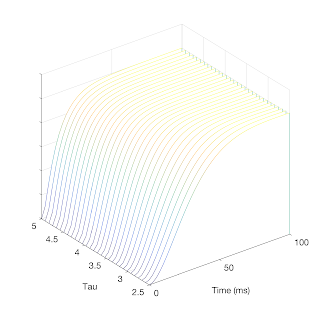Similarly as we sweep $\tau$ there is also only a small change in the simulated closed loop performance.

Having this type of invariance has the benefit that once some more serious pilots and tweakers find a set of costs that work well for them it should apply more -- both when using them on different frames and sharing them amongst other pilots.

### Real world results

As usual when testing these kind of control algorithm, I threw Freedom on a frame, which is a flight controller that has an Overo Gumstix on it that lets me log all the sensor data in the flight. I can then analyze the filter offline (as well as reprocess the data to refine things like the filter).

Here it is tied up to isolate one axis so I could test extremely high rotational speeds that I couldn't otherwise indoors.

A video posted by peabody124 (@peabody124) on

And from that I can download the logs and look at the performance when doing fast movements in attitude mode as well as in rate control.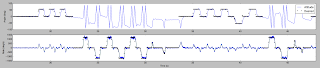Here you can see me switching between rate and attitude control. In both cases the responses are extremely crisp.

Here is some data from a free flying frame.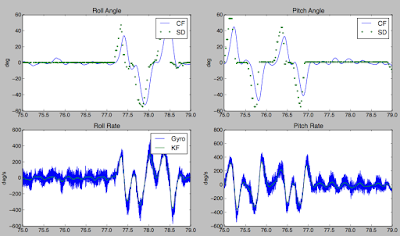The green points in the top trace show the desired angle and the blue curve is the actual angle. The system responds very promptly to the input stimuli. The bottom trace again shows the estimator that again smooths the gyro down.

This graph shows that the system responds very promptly. Because of the smoothed gyro and torque estimates, LQG can tolerate much greater effective gains than can work with PID control and no smoothing.

## So why LQG?

After going through all this, it is worth taking a moment to ask why do we want to implement this. There are two main benefits corresponding to the two halfs of LQG.

The first part is the benefit of the estimation component. By running the Kalman filter we fuse together the inputs that we are sending to the motor and the noisy gyro estimates to get the best possible estimate of what is really going on. Superficially, this has the benefit of significantly denoising the gyro signal -- after all that very high frequency noise is physically implausible but applying a low pass filter to it introduces latencies that people are always trying to chase out. In addition, it allows us to directly estimate the instantanous (normalized) torque being generated by the motors. This comes from the latency of their responses and allows us to properly damp the control algorithm rather than the ad hoc derivative term typical in PID. The derivative is critical for good performance and those who have been in this game long enough to remember when the bandlimited derivative from APM was ported over to OpenPilot recall how much of a performance boom it was. However, that band limiting is required because of the amount of noise when directly taking the difference of gyro measurements. The state estimation reduces the amount of noise there allowing much greater derivative terms. When looking at the parameters as the corresponding PID terms we can use much much higher PID parameters when using the state estimator. In practice I'm using a factor of 10 higher values than without this estimator.

The second half is the actual calculation of the gains. As I referenced in the beginning after we got the system identification going I came up with some analytic equations to optimize the PID coefficients from this (derivation is here). This has worked well and feedback on Tau Labs autotuning  has always been quite positive. However, LQR is a much more principled and mainstream approach to the same problem. It is more computationally intensive, but by only having to calculate the DARE equation once given the system identification parameters, we can do this before take off and avoid this cost in real time. Thus the costs of running this LQG is essentially the same as PID.

## Conclusion

LQG is a more modern control scheme than PID. It flies much better. This implementation simply requires a regular autotune flight, but instead of using the math I previously developed that is now used by TauLabs/LibrePilot/Dronin it directly computes LQR coefficients. Here I show it flying on 3 frames of much different sizes without issue:

#### 13 comments:

1.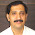Control system are important and are present almost everywhere in our daily lives. Control system provides an output or response for a given input or stimulus.
Transformer Manufacturer in India

2.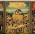Excellent. Any chance of this making it into the flight code in the near future?

1.OK I see it in gitHub.

3.Glowtape from IRC here...

I've implemented the math as a plain rate controller (no angle component) over this weekend, and by chance I got a dry day today.

I had to spend quite a while in MATLAB to figure out Q and R for the RTKF. Same for Q for the LQR, for trying to get sane step responses. Especially latter seems confusing, due to the difference in magnitudes. My current Q for rate is 0.00001 and the Q for torque is 0.5*non-exp Beta (e.g. 0.5*10.77 for one axis).

Despite working OK in the living room with simple hover and actuations, I figured this can't be right.

But then I had a bout of sunshine today and went outside. Like holy f%@#, this works so damn well. Especially extreme manoeuvers like flips, it stops on a dime.

Awesome work!

4.Our Academic Essay Writing service is offered to you so that you don’t have to struggle with your academic homework. If you are depressed then you shouldn’t be anymore because you have us with you. You have spent hours and hours but you still cannot come up with something to write in your homework. Let us assure you that this happens a lot. You cannot just punch the wall if your academic homework is still pending. If you do punch it then you should be ready to face the wrath of your mom. In this kind of situation, you can get scolded for hours or you can pull up a smart move by choosing our academic essay writing service. We have the experience so you don’t have to worry about the quality of your academic essay. It has been more than a decade since we have been working and assisting students with their homework. We have satisfied countless of students and we are still working to please them. We work on countless of orders every day. However, we don’t lose the will and conduct the operations with as much motivation. You can entrust our academic essay writers with your homework and they will deliver it when it is completed.

It is actually the quality of the academic essay writing service which has made us popular in the world. Our services are made in such a way that you don’t have to go through a long process in order to get our cheap writing service. Our services are made in a very simplistic way and everything about us is explained on our website. You can check our cheap academic essay writing service and see if everything we told you was the truth or not. You get the help of the best academic essay writers if you choose us. We have gained the satisfaction of thousands of students. You can see the rating on our website. To provide you the best quality, we have hired the experts who are highly qualified. They have the experience and the skills which are required to ensure good quality essay. You can simply ask our experts online anything concerning the essay and they will debrief you properly. Along with quality, there are many other aspects and packages which we offer you for your satisfaction. https://liveassignmenthelp.com
https://topacademictutors.com/academic-essay-writing/

5.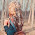6.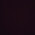Sample Assignment is a renowned for assignment help in Australia and has assisted thousands of international students with their academics. Our dedicated team of experts has been providing full-fledged assignments to students pursuing their courses at various colleges and universities, and found to be avidly googling "assignment help Sydney" across the continent. While Australia is among the most preferred destinations for individuals from around the world, Assignment Experts has also won the trust of a vast pool of students here. The Australian Assignment help, such as Sample Assignment, can be easily contacted via WhatsApp and Messenger too. With our 24-hour online academic assistance, any student can reach out to us whenever he or she is in the need of help of a subject expert.

7.For nursing students, it gets a bit difficult to even make time to content an online assignment help providing company for nursing assignment help. Which is why, we, at Online Assignment Expert have extended our assignment help services which only exist in order to make it more convenient for the nursing students to fetch assignment solutions from an online assignment help company. Therefore, in order to avail our nursing assignment help offering no plagiarism, Free Turnitin, Partial Payment, Unlimited Revisions, etc. You can contact our team of highly professional, experienced, PhD experts to avail the discounted services!

8.Hey, do check out our Cryptography assignment help provided by the subject experts at TutorVersal. We are an online assignment help provider who assists students in overcoming their assignment writing challenges. Our highly qualified team of academic helpers write quality assignments that are 100% plagiarism-free and deliver them right on time. Recently, we launched child care assignment help service, and it has got a favorable response from hundreds of students in Australia who used it. We provide assignment solutions for over 180 subjects such as management, economics, nursing, engineering, and more. You can easily get your essays, dissertations, and case study assignments solved by us and score excellent grades in them!

9.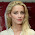Hello. I appreciate your concern. However, there are numerous Australian assignment help service provider in Australia that are offering their professional assistance to students in their academic assignments.
Though, I would like to suggest you about an assignment writing service provider that goes by the name My Assignment Help Oz. MAHOZ consist of a specialised team of experts who knows everything about marking rubrics and formats. Also, the team of experts are PhD holders from reputed universities in Australia and knows every microscopic detail about their respective course study.
Thus, they can provide students with best Australian Assignment help services.
In addition, the team of writers claims to be best in preparing: essays, reports, articles, dissertations, theses, case study etc. They also acquire knowledge in various subjects like management, nursing, accounting, psychology, engineering etc.
Thus, all who availed their services are pretty much satisfied and mostly all the students are very happy with the kind of quality solutions that they prepare, well-before stipulated deadlines, making them prime Australian assignment help provider.

10.Hello, it is a great write-up that, I am sure, will add a lot of value to the students and their assignment writing process. Laden with well researched content, the students will get a lot of things to learn. And the students who are looking for a reliable and cheap assignment help Australia service, My Assignment Services is the destination for them. I am academic expert having more than 10 years of industry experience and 5 years of assignment expert. I know what it takes to write an assignment that has the ability to fetch high grades. Our experts know how to make a boring assignment question unique and original. This is why we are the number one assignment provider for all university going students.

11.Concursos públicos no Brasil. Concursos Abertos, previstos 2019 e concurso em Andamento. Confira Edital, Simulados e Provas de concursos anteriores. Entre em contato com o Concursos para mais informações. concursos abertos 2018, Lista de Concursos Públicos no Brasil com inscrições abertas em todo o país. Confira a lista de todos os pci concursos abertos no país em e 2018.

12.This is really awesome, am so glad to read this informative article.
Thanks for sharing.

Custom Packaging Boxes USA